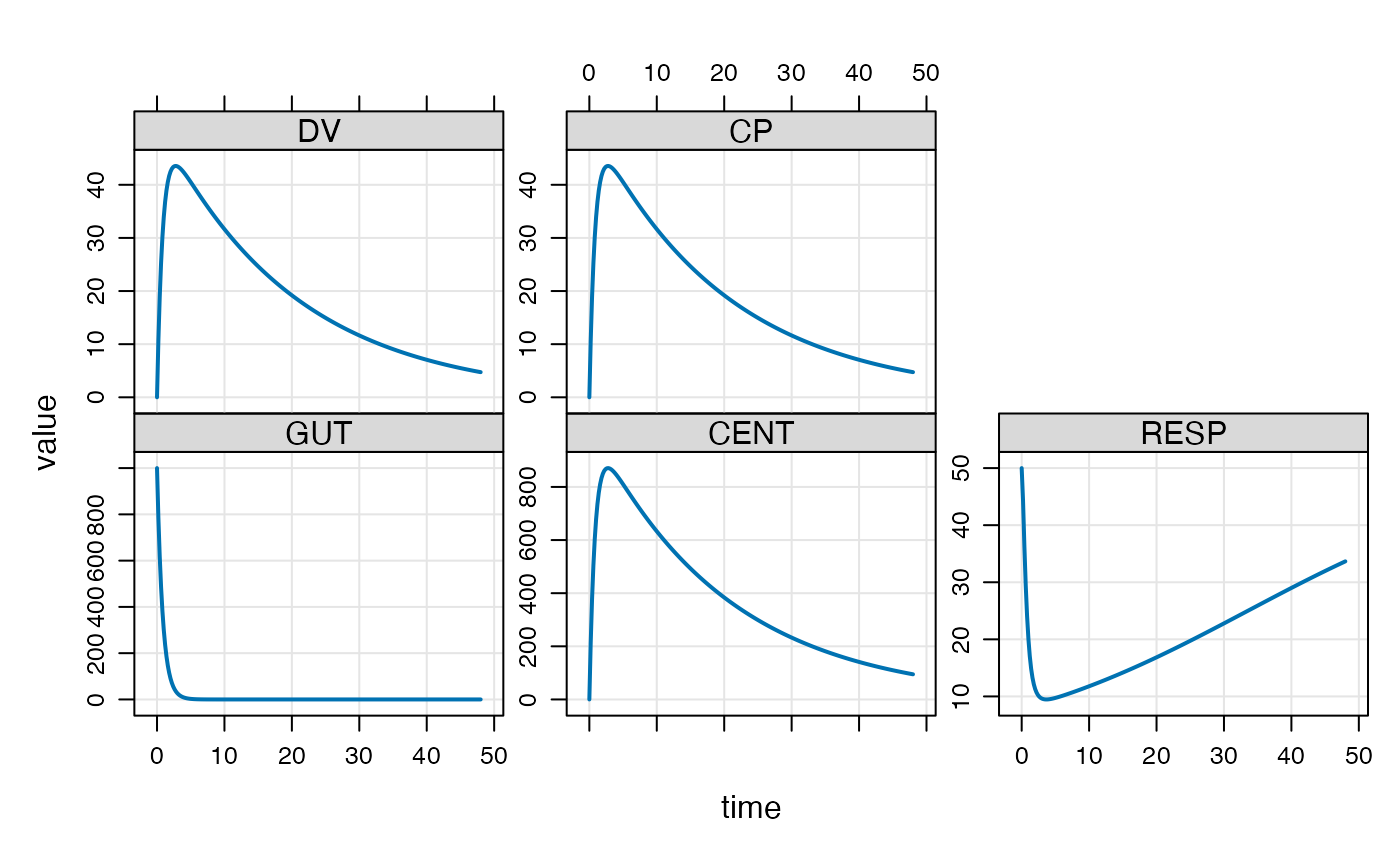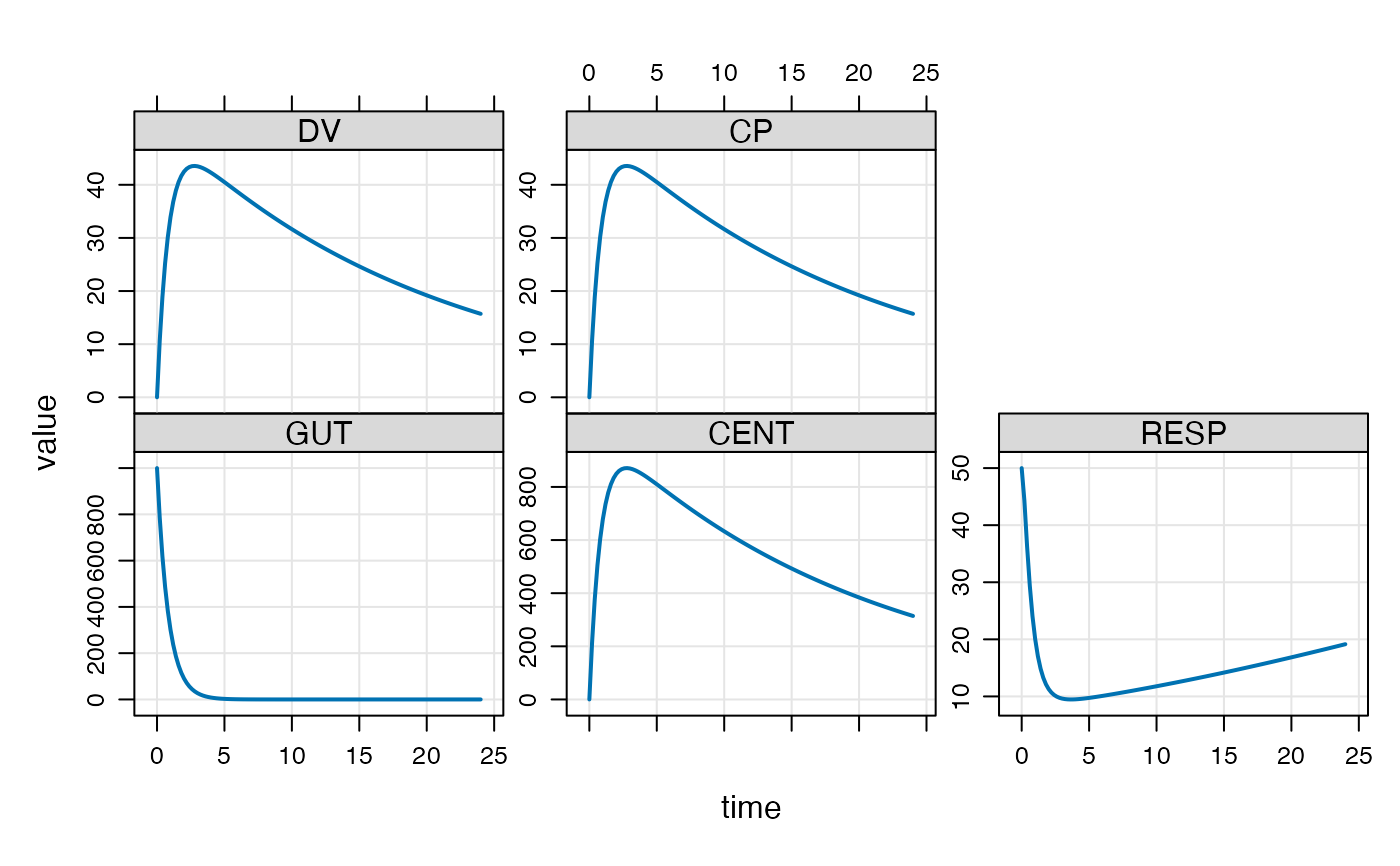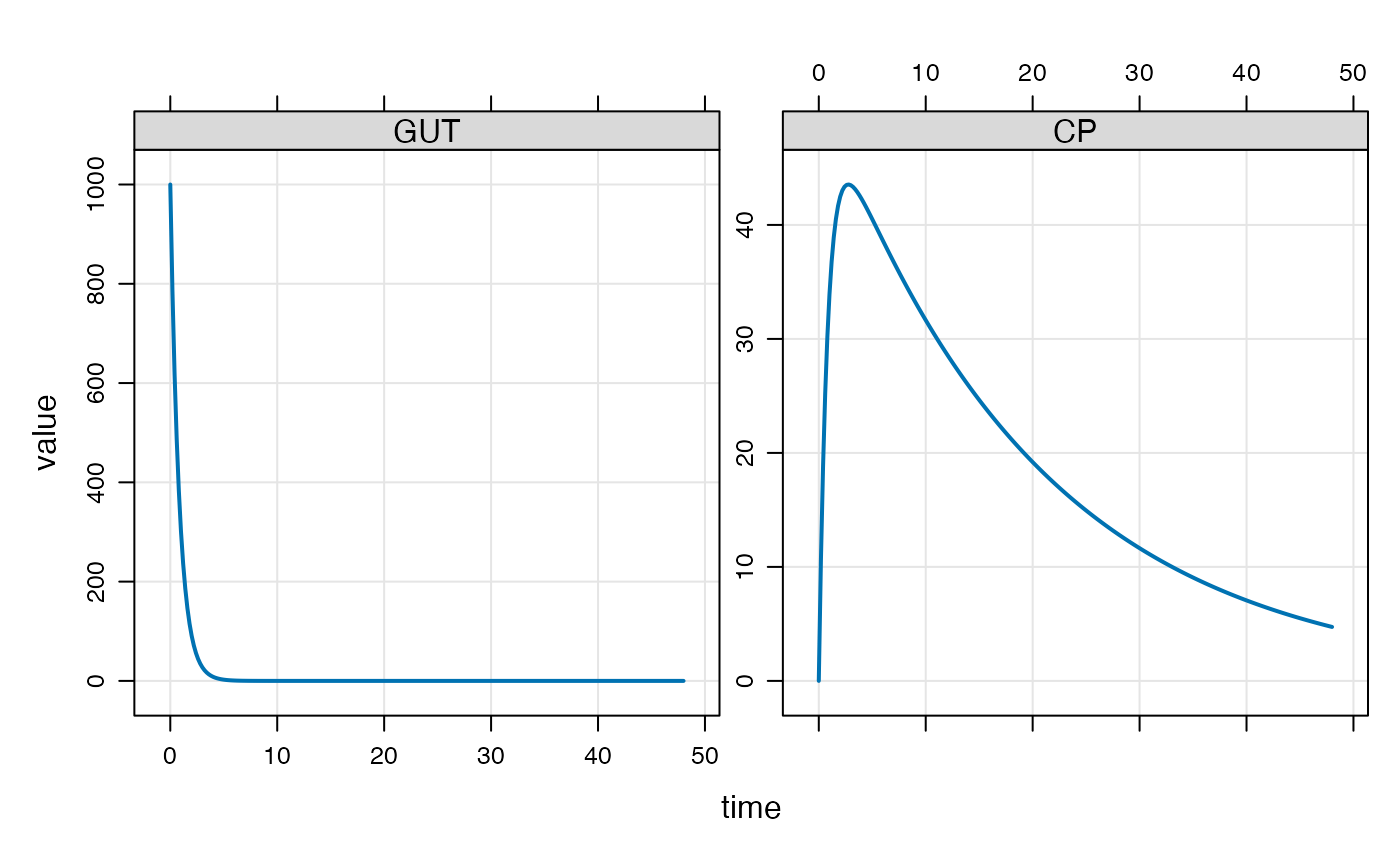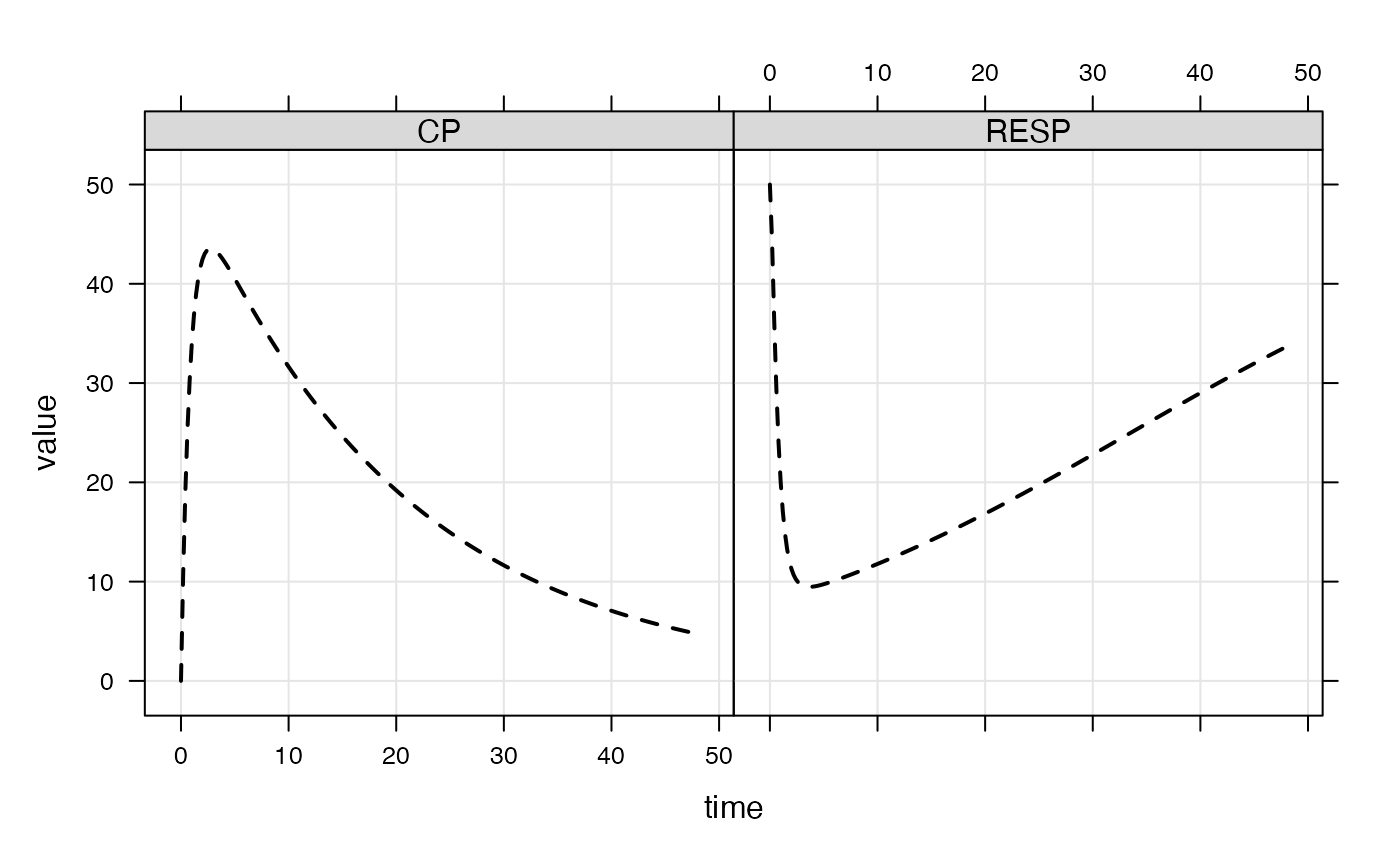Generate a quick plot of simulated data

## Usage

# S4 method for mrgsims,missing
plot(x, limit = 16, ...)

# S4 method for mrgsims,formula
plot(
x,
y,
limit = 16,
show.grid = TRUE,
outer = TRUE,
type = "l",
lwd = 2,
ylab = "value",
groups = ID,
scales = list(y = list(relation = "free")),
logy = FALSE,
logbr = 1,
...
)

# S4 method for mrgsims,character
plot(x, y, ...)

## Arguments

x

mrgsims object

limit

limit the the number of panels to create

...

other arguments passed to xyplot

y

formula used for plotting

show.grid

logical indicating whether or not to draw panel.grid

outer

passed to xyplot

type

passed to xyplot

lwd

passed to xyplot

ylab

passed to xyplot

groups

passed to xyplot

scales

passed to xyplot

logy

plot the y variables on log scale

logbr

log scale breaks indicator; use 1 for breaks every log unit; use 3 for breaks every half log unit; use 0 for default breaks

## Examples


mod <- mrgsolve::house(end=48, delta=0.2) %>% init(GUT=1000)

out <- mrgsim(mod)

plot(out)plot(out, subset=time <=24)plot(out, GUT+CP~.)plot(out, CP+RESP~time, col="black", scales="same", lty=2)if (FALSE) {
plot(out, "CP RESP, GUT")
}